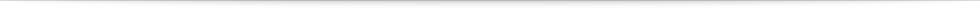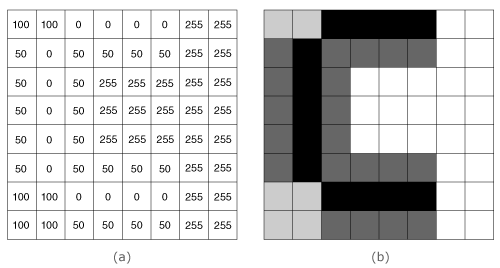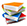Teach
With
Bigshot
 Prev Introduction      Pixel      Image Readout      Color Sensing Color      Illustrations Next### Image Sensor: A Grid of Pixels

An image sensor is usually a two-dimensional grid of pixels. The number of pixels on the grid is called the resolution of the image sensor. Bigshot has a 3 megapixel sensor, which means it has about 3 million pixels (arranged in a grid of 1536 rows and 2048 columns).

Figure 3 shows a small (3×4) grid of pixels − each with its own microlens and circuitry − connected to an external read-out circuit. Each pixel generates a voltage (measured in volts) that is proportional to the light energy falling on the pixel. The voltages of the entire grid of pixels are read out one pixel at a time and converted to numbers by the analog-to-digital converter (ADC) . Press the play button at the top-left to see the read-out process in action.Figure 4: Digital and visual representations of an image

The end result of the read-out process is a two-dimensional array of numbers that is called a digital image. Each number represents the light energy falling at the corresponding pixel. In an 8-bit sensor, black is 0, white is 255, and all the numbers in between are shades of gray. In Figure 4, a digital image is shown as an array of numbers on the left and brightness levels on the right.

The pixels we have described above can only measure the brightness of the light falling on them − they are color blind. Now, let's take a look at how pixels can measure color.

 Prev Next###References

  "Analog-to-digital converter," [Online]. Available: http://en.wikipedia.org/wiki/Analog-to-digital_converter. [Accessed: Jun 4, 2012].